# Fluid Mechanics: Bernoulli & Energy Equations Problem- Help?

Hello there. I took a test yesterday in my thermal fluids 1 class and I was hoping somebody could confirm or critique my answer. (Don't worry, I asked the professor first if I could take the picture)

The problem is:
Water is pumped from a lake to a pool through a 0.05 m diameter pipe. System friction losses are hf = 50V2/(2g), where V is the average velocity in the pipe. If the power of the pump is 10 kW, what is the mass flow rate pumped?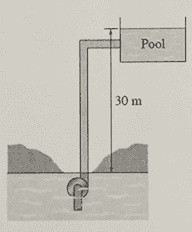The diagram given with the problem is attached as well as the two pages of my work. I was fairly certain that I messed up on the first page so I redid it on the second page and hope that it was the correct approach. Thermodynamics & Fluid Mechanics are tough for me because you really have to know exactly how, when, why, and which formulas to use at precisely the correct time. If somebody could just look over my work and let me know if I was on the right track, what my errors were, and/or the correct approach, I'd really appreciate it!

Thanks,
H.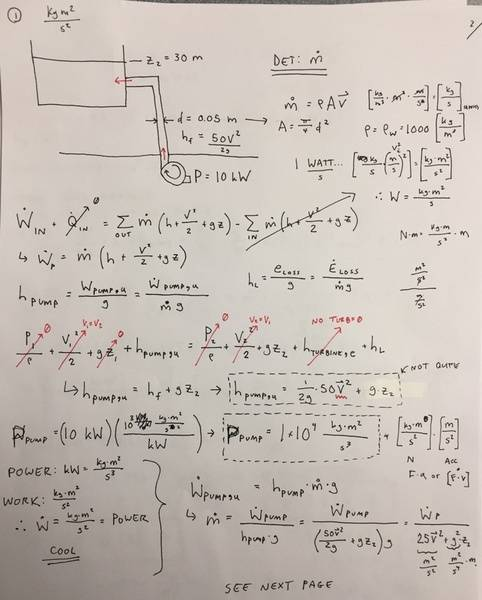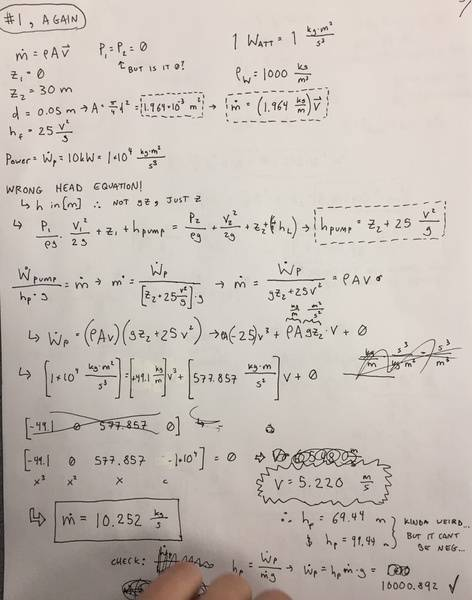Chestermiller
Mentor
It looks like you have been trying to use two versions of an equivalent equation:

1. The open system (control volume) version of the first law of thermodynamics (page 1)
2. The Bernoulli equation, modified to include pump work and friction energy losses (page 2)

Given that the friction loss is given with a g in the denominator, my inclination is that they meant for you to use method 2. For the Bernoulli version, I recommend using point 1 before the inlet of the pump (so p1=0 and v1 = 0) and point 2 (after the outlet in the pool (so p2=0 and v2 = 0); so,
$$h_{pump}=(z_2-z_1)+h_L$$
This is what you ended up with, and, given the rest is straightforward, it looks like you did it right.

•berkeman and hdp12
This is what you ended up with, and, given the rest is straightforward, it looks like you did it right.

That is very good news! They take forever grading the tests and I just really wanted some positive feedback. If it's not too much to ask, would you tell me if I did the second question correctly too? There are only 2 questions so I promise this is the last one!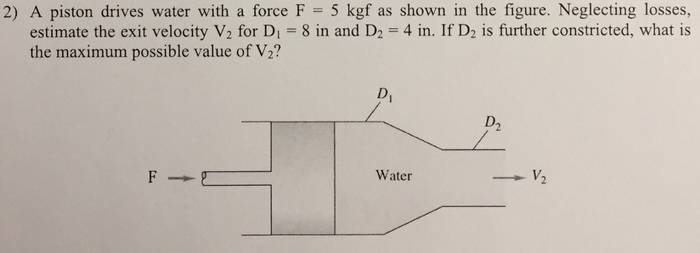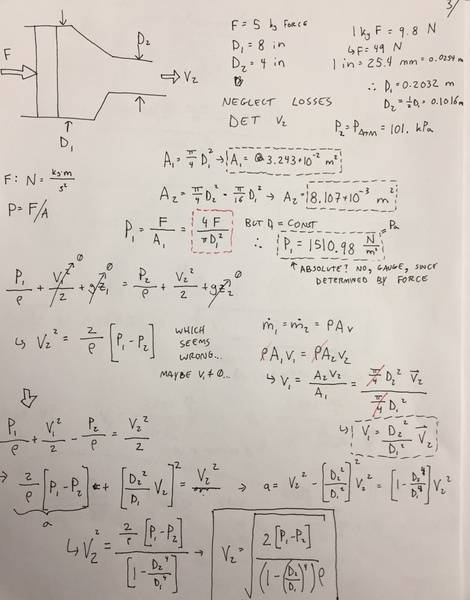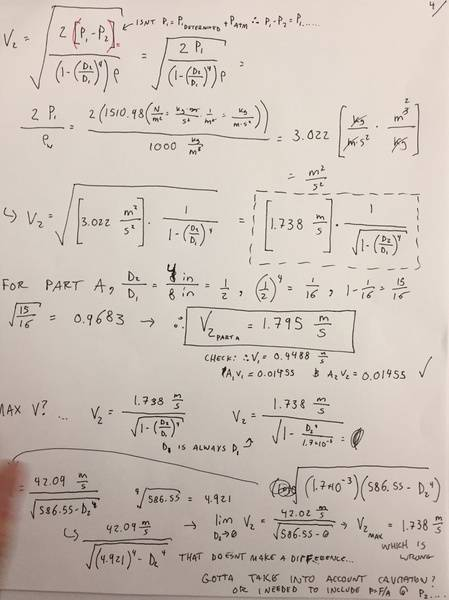Chestermiller
Mentor
That is very good news! They take forever grading the tests and I just really wanted some positive feedback. If it's not too much to ask, would you tell me if I did the second question correctly too? There are only 2 questions so I promise this is the last one!Please start a new thread for this. Also, please put it into Engineering and Computer Science homework forum, and use the homework template. Thanks.